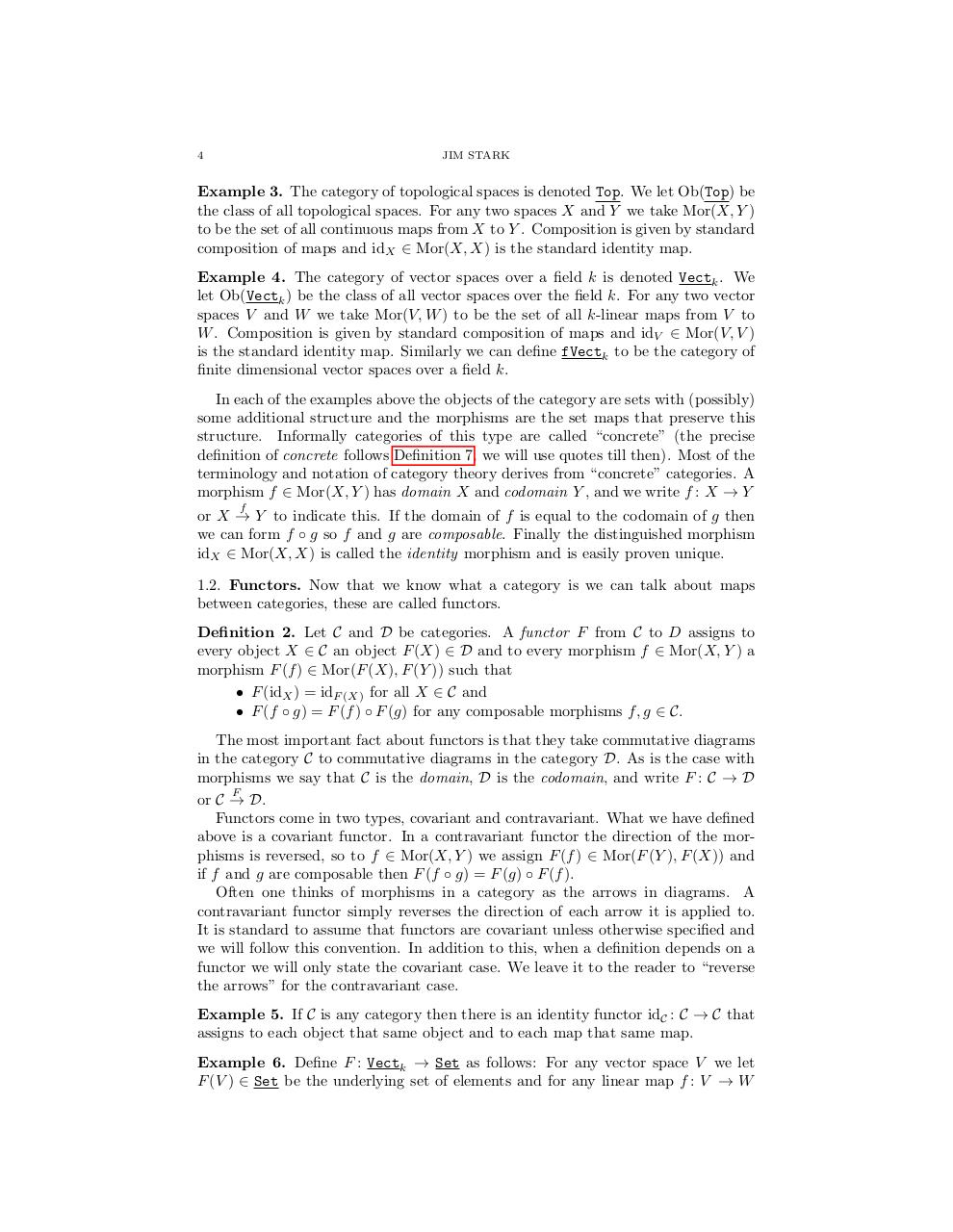# CategoryTheory.pdfPage 1 2 3 45619

#### Text preview

4

JIM STARK

Example 3. The category of topological spaces is denoted Top. We let Ob(Top) be
the class of all topological spaces. For any two spaces X and Y we take Mor(X, Y )
to be the set of all continuous maps from X to Y . Composition is given by standard
composition of maps and idX ∈ Mor(X, X) is the standard identity map.
Example 4. The category of vector spaces over a field k is denoted Vectk . We
let Ob(Vectk ) be the class of all vector spaces over the field k. For any two vector
spaces V and W we take Mor(V, W ) to be the set of all k-linear maps from V to
W . Composition is given by standard composition of maps and idV ∈ Mor(V, V )
is the standard identity map. Similarly we can define fVectk to be the category of
finite dimensional vector spaces over a field k.
In each of the examples above the objects of the category are sets with (possibly)
some additional structure and the morphisms are the set maps that preserve this
structure. Informally categories of this type are called “concrete” (the precise
definition of concrete follows Definition 7, we will use quotes till then). Most of the
terminology and notation of category theory derives from “concrete” categories. A
morphism f ∈ Mor(X, Y ) has domain X and codomain Y , and we write f : X → Y
f

or X → Y to indicate this. If the domain of f is equal to the codomain of g then
we can form f ◦ g so f and g are composable. Finally the distinguished morphism
idX ∈ Mor(X, X) is called the identity morphism and is easily proven unique.
1.2. Functors. Now that we know what a category is we can talk about maps
between categories, these are called functors.
Definition 2. Let C and D be categories. A functor F from C to D assigns to
every object X ∈ C an object F (X) ∈ D and to every morphism f ∈ Mor(X, Y ) a
morphism F (f ) ∈ Mor(F (X), F (Y )) such that
• F (idX ) = idF (X) for all X ∈ C and
• F (f ◦ g) = F (f ) ◦ F (g) for any composable morphisms f, g ∈ C.
The most important fact about functors is that they take commutative diagrams
in the category C to commutative diagrams in the category D. As is the case with
morphisms we say that C is the domain, D is the codomain, and write F : C → D
F
or C → D.
Functors come in two types, covariant and contravariant. What we have defined
above is a covariant functor. In a contravariant functor the direction of the morphisms is reversed, so to f ∈ Mor(X, Y ) we assign F (f ) ∈ Mor(F (Y ), F (X)) and
if f and g are composable then F (f ◦ g) = F (g) ◦ F (f ).
Often one thinks of morphisms in a category as the arrows in diagrams. A
contravariant functor simply reverses the direction of each arrow it is applied to.
It is standard to assume that functors are covariant unless otherwise specified and
we will follow this convention. In addition to this, when a definition depends on a
functor we will only state the covariant case. We leave it to the reader to “reverse
the arrows” for the contravariant case.
Example 5. If C is any category then there is an identity functor idC : C → C that
assigns to each object that same object and to each map that same map.
Example 6. Define F : Vectk → Set as follows: For any vector space V we let
F (V ) ∈ Set be the underlying set of elements and for any linear map f : V → W